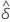# Methods and formulas for test for 2x2 tables for Cross Tabulation and Chi-Square

Select the method or formula of your choice.

## Fisher's exact test

Fisher's exact test is a test of independence. The test is based on an exact distribution rather than on the approximate chi-square distribution used for the Pearson's and likelihood ratio tests. Fishers exact test is useful when the expected cell counts are low and the chi-square approximation is not very good.

### Formula

The p-value is based on a hypergeometric distribution with the following parameters:
population size
total number of observations
number of successes in the population
number of observations in the first row
sample size
number of observations in the first column
The p-value is for a two-sided alternative. The computation is the sum of the hypergeometric probabilities over the values from 0 to the population size that are less than or equal to the probability of the observed value.

## McNemar's exact test

McNemar's test compares proportions that are observed before and after a treatment. For example, you can use McNemar's test to determine whether a training program changes the proportion of participants who correctly answer a question.

Observations for McNemar's test can be summarized in a two-by-two table, as shown below.

 After Treatment Before Treatment Condition True Condition Not True Total Condition True n11 n12 n1. Condition Not True n21 n22 n2. Total n·1 n·2 n··

The condition for the training example is a correct answer. Thus, n21 represents the number of participants who answer the question correctly after training but not before training. And n12 represents the number of participants who answer the question correctly before training but not after training. The total number of participants is represented by n...

### Estimated difference

Let δ be the difference between the marginal probabilities, p1.- p.1, in the population. The estimated difference,, is given by the following formula:

### Confidence interval

An approximate 100(1 – α)% confidence interval is given by the following formula:

where α is the significance level for the test, z α/2 is the z-score associated with a tail probability of α/2, and SE is given by the following formula:

### P-value

The null hypothesis is δ = 0. The exact p-value for the test of the null hypothesis is calculated as:

where X is a random variable that is drawn from a binomial distribution with an event probability of 0.5 and a number of trials equal to n21 + n12.

## Cochran-Mantel-Haenszel test

The test assumes no three-way interaction exists. The purpose of the test is to assess the degree of relationship between two dichotomous variables while controlling for a nuisance variable. The CMH statistic is compared with a chi-square percentile with one degree of freedom.

The Cochran-Mantel-Haenszel (CMH) test applies only if three or more classification variables exist, and the first two variables have two levels each. All variables beyond the first two are treated as a single variable Z for the purposes of the CMH test, with each combination of levels treated as a level of Z.

### Notation

TermDescription
klevel of Z
n11k number of observations in first row, first column
n1+k number of observations in first row
n+1k number of observations in first column
n++k total number of observations
n2+k number of observations in second row
n+2k number of observations in second column
By using this site you agree to the use of cookies for analytics and personalized content.  Read our policy